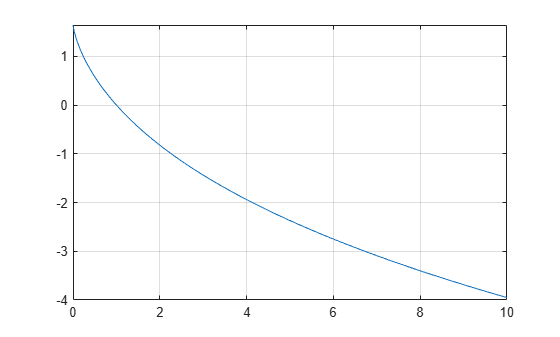# dilog

Dilogarithm function

## Syntax

``dilog(X)``

## Description

example

````dilog(X)` returns the dilogarithm function.```

## Examples

### Dilogarithm Function for Numeric and Symbolic Arguments

Depending on its arguments, `dilog` returns floating-point or exact symbolic results.

Compute the dilogarithm function for these numbers. Because these numbers are not symbolic objects, `dilog` returns floating-point results.

`A = dilog([-1, 0, 1/4, 1/2, 1, 2])`
```A = 2.4674 - 2.1776i 1.6449 + 0.0000i 0.9785 + 0.0000i... 0.5822 + 0.0000i 0.0000 + 0.0000i -0.8225 + 0.0000i```

Compute the dilogarithm function for the numbers converted to symbolic objects. For many symbolic (exact) numbers, `dilog` returns unresolved symbolic calls.

`symA = dilog(sym([-1, 0, 1/4, 1/2, 1, 2]))`
```symA = [ pi^2/4 - pi*log(2)*1i, pi^2/6, dilog(1/4), pi^2/12 - log(2)^2/2, 0, -pi^2/12]```

Use `vpa` to approximate symbolic results with floating-point numbers:

`vpa(symA)`
```ans = [ 2.467401100272339654708622749969 - 2.1775860903036021305006888982376i,... 1.644934066848226436472415166646,... 0.97846939293030610374306666652456,... 0.58224052646501250590265632015968,... 0,... -0.82246703342411321823620758332301]```

### Plot Dilogarithm Function

Plot the dilogarithm function on the interval from 0 to 10.

```syms x fplot(dilog(x),[0 10]) grid on```### Handle Expressions Containing Dilogarithm Function

Many functions, such as `diff`, `int`, and `limit`, can handle expressions containing `dilog`.

Find the first and second derivatives of the dilogarithm function:

```syms x diff(dilog(x), x) diff(dilog(x), x, x)```
```ans = -log(x)/(x - 1) ans = log(x)/(x - 1)^2 - 1/(x*(x - 1))```

Find the indefinite integral of the dilogarithm function:

`int(dilog(x), x)`
```ans = x*(dilog(x) + log(x) - 1) - dilog(x)```

Find the limit of this expression involving `dilog`:

`limit(dilog(x)/x, Inf)`
```ans = 0```

## Input Arguments

collapse all

Input, specified as a symbolic number, variable, expression, or function, or as a vector or matrix of symbolic numbers, variables, expressions, or functions.

collapse all

### Dilogarithm Function

There are two common definitions of the dilogarithm function.

The implementation of the `dilog` function uses the following definition:

`$\text{dilog}\left(x\right)=\underset{1}{\overset{x}{\int }}\frac{\mathrm{ln}\left(t\right)}{1-t}\text{\hspace{0.17em}}dt$`

Another common definition of the dilogarithm function is

`${\text{Li}}_{2}\left(x\right)=\underset{x}{\overset{0}{\int }}\frac{\mathrm{ln}\left(1-t\right)}{t}\text{\hspace{0.17em}}dt$`

Thus, dilog(x) = Li2(1 – x).

## Tips

• `dilog(sym(-1))` returns ```pi^2/4 - pi*log(2)*i```.

• `dilog(sym(0))` returns `pi^2/6`.

• `dilog(sym(1/2))` returns ```pi^2/12 - log(2)^2/2```.

• `dilog(sym(1))` returns `0`.

• `dilog(sym(2))` returns `-pi^2/12`.

• `dilog(sym(i))` returns ```pi^2/16 - (pi*log(2)*i)/4 - catalan*i```.

• `dilog(sym(-i))` returns ```catalan*i + (pi*log(2)*i)/4 + pi^2/16```.

• `dilog(sym(1 + i))` returns ```- catalan*i - pi^2/48```.

• `dilog(sym(1 - i))` returns ```catalan*i - pi^2/48```.

• `dilog(sym(Inf))` returns `-Inf`.

 Stegun, I. A. “Miscellaneous Functions.” Handbook of Mathematical Functions with Formulas, Graphs, and Mathematical Tables. (M. Abramowitz and I. A. Stegun, eds.). New York: Dover, 1972.

## Support

#### Mathematical Modeling with Symbolic Math Toolbox

Get examples and videos